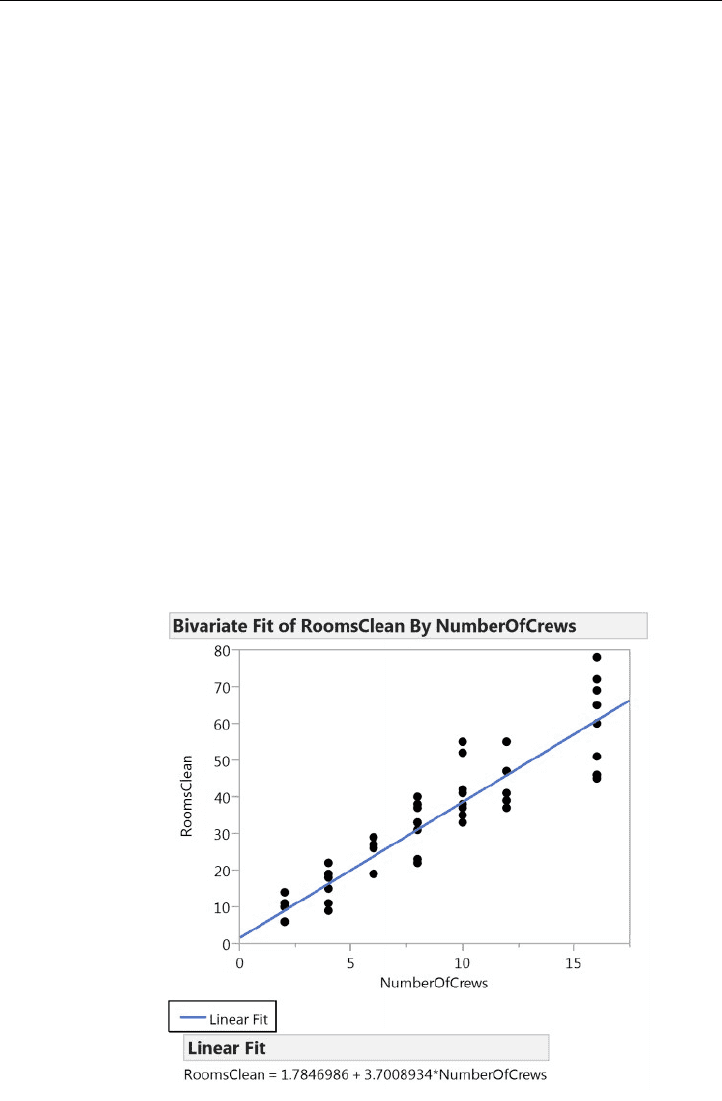Class Notes (1,100,000)
US (490,000)
UPenn (2,000)
STAT (70)
Lecture 23

# STAT 101 Lecture Notes - Lecture 23: Mean Squared ErrorPremium

Department
Statistics
Course Code
STAT 101
Professor
Richard Waterman
Lecture
23

This preview shows half of the first page. to view the full 3 pages of the document.Stat 101 - Introduction to Business Statistics - Lecture 23: Line of Fit
Reasons to fit a line through data
Graphically summarize
● Interpolate
Forecast/extrapolate (with caution)
Mathematically leverage the equation: derivatives and optimizations
Least Squares
the best line minimizes the sum of the squares of the vertical distances from the point to
the line, and is called the Least Squares Line
Sometimes, we may fit a line on a transformed scale, then back-transform, which gives
best fitting curves
The difference between y and yhat (y - yhat) is called the residual (e)
Fitted values given as:
yhat = b0 + b1x
○ b1 = r (sy / sx ) , where r is the correlation between y & x
○ b0 = ybar - (b1 * xbar)
Graph the line:
Slope interpretation: the change in y for every one unit change in x